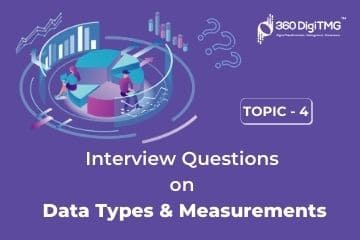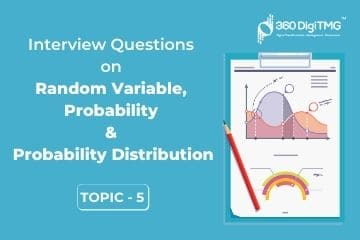Home / Blog / Interview Questions on Data Science / Data Type and Measurements

# Data Type and Measurements

• September 29, 2020
• 3801
• 21### Meet the Author : Mr. Bharani Kumar

Bharani Kumar Depuru is a well known IT personality from Hyderabad. He is the Founder and Director of Innodatatics Pvt Ltd and 360DigiTMG. Bharani Kumar is an IIT and ISB alumni with more than 17 years of experience, he held prominent positions in the IT elites like HSBC, ITC Infotech, Infosys, and Deloitte. He is a prevalent IT consultant specializing in Industrial Revolution 4.0 implementation, Data Analytics practice setup, Artificial Intelligence, Big Data Analytics, Industrial IoT, Business Intelligence and Business Management. Bharani Kumar is also the chief trainer at 360DigiTMG with more than Ten years of experience and has been making the IT transition journey easy for his students. 360DigiTMG is at the forefront of delivering quality education, thereby bridging the gap between academia and industry.

1. ## What is Nominal Data?

Name of categories (there is no natural order among categories).

There is no inherent order and has a limited set of entries.

Usually, nominal data is either alphabetical (string) or in text format.

Nominal data has to be converted into a dummy variable encoding format for ML algorithms to understand the same.

Eg: Color names, Gender, Brand names, Genre Labels, etc.

We can perform only 'Count' as a mathematical operation.

2. ## What is Ordinal Data?

Categories that have particular order (Inherent order).

Ordinal data has to be converted to its numeric equivalent using encoding techniques.

Eg: Shirt size: S, M, L, XL, XXL; Gate numbers in the airport: 1, 2, 3, 4.....

The difference in the different levels or values of the ordinal data is consistent in direction and consistency need not be in magnitude.

The difference in the different levels has no meaning.

We can perform 'Count' as well as 'Rank' the items in an order.

3. ## What is Interval Data?

Interval scales are the numeric scales where we know both the order of the values along with the exact differences between the values.

The difference between the levels has a meaningful rationale.

No natural zero (Absence of absolute zero). This means, if the temperature is zero, it does not mean there is no temperature.

Eg: Time, Temperature, Date, and IQ level.

We can perform mathematical operations - Addition & Subtraction

4. ## What is the Ratio?

Ratio data is very much like the interval data – the values must be numerical where the difference between points is standardized and quite meaningful.

Whereas, in order for data to be considered as the ratio data, it must have a true zero value, which means ratio data cannot have negative values.

Eg: Height, Weight, etc. If we have zero money then it means there is no money.

We can perform mathematical operations such as Addition, Subtraction, Multiplication, and Division.5. ## What is a Factor?

The factor is a variable, which can take a limited set of values. For example: 'Gender' is a variable that can take two levels - 'Male' & 'Female'.

Another example is 'Month', which can take '12' levels - Jan, Feb, Mar,....., Dec.

6. ## What are the broad classifications of data types?

Broadly speaking data can be classified as Continuous data and Discrete data.

Discrete data can be further classified as Categorical data and Count data.

Categorical data is further classified as Binary categorical data and Multiple categorical data.

Multiple categorical data is further classified as Nominal data and Ordinal data.

Continuous data & Count data are considered Quantitative data, whereas Categorical data is considered as Qualitative data.

7. ## What is the difference between structured, semi-structured and unstructured data?

Structured data: Data, which can be arranged in a neat tabular format with rows and columns is called as structured data.

Examples of the same include RDBMS, SQL, MySQL, Oracle DB, MS SQL, etc.

Unstructured Data: Data, which cannot be arranged in a tabular format or in its raw format is called as unstructured data.

Examples of the same include Videos, Images, Audio, Textual, etc.

Unstructured data can be transformed into structured data by applying a few statistical techniques.

Semi-Structured Data: Data, which is neither unstructured and nor is it structured and lies somewhere midway is called as semi-structured data.

Examples of the same include XML, JSON, HTML, etc.

8. ## What is the difference between Big Data and Non-Big Data?

Big Data is that data, which cannot be stored and/or which cannot be processed using traditional storage and hardware/software.

Big Data is majorly characterized by 5 Vs - Velocity, Veracity, Volume, Variety, Value.

Non-Big Data is that data, which can be stored and processed using traditional hardware/software.

9. ## What is the difference between Cross-Sectional data, Time Series data and Longitudinal data/Panel data?

Data where the sequence based on data & time is unimportant, is called as cross-sectional data. This data usually contains multiple variables. Eg: Data where variables includes age, income, gender, etc., and based on that we want to predict the loan defaulters.

Data where the sequence based on data & time is important, is called as time-series data. This data usually contains single variable. Eg: Predicting sales for example includes only one variable called sales and it will have monthly, weekly, daily sales, which will be in a sequence.

Data where the sequence based on data & time is important & contains multiple variables is called as longitudinal data or panel data. Eg: Predicting sales across various countries is an example of longitudinal data or panel data.11. ## What is the difference between balanced and imbalanced/rare datasets?

Categorical Data (Binary): Data where one class representation is less than 30% is called as imbalanced dataset. 30% is a generic thumb rule. Eg: Output variable has Default or Not Default details. 29% of the data in output variable says default and another 71% says not default.

Categorical Data (Multiple): Data where count or percentage of one of the classes is significantly less or more than the other classes. Eg: Output variable has 0, 1, 2, 3....9, handwritten digits and algorithm has to recognize the handwritten digits. If one of the classes '1' has only 2% of representation and if another class '10' has 10% representation then it is imbalanced dataset.

Continuous: If the dataset is bimodal or non-normal then it may be one case of imbalanced dataset.

12. ## What is the difference between offline processing and online processing?

Offline processing means data is processed offline without need for internet connection. Here data is usually processed in batches, which is called as Batch processing.

Online processing means data is processed online and internet connection is needed. As and how data arrives, it is processed and it is called as streaming data or real-time processing.

13. ## What is a Random Variable?

Any variable whose output varies and has a chance associated with the output values is called as Random variable. Eg: Flipping a coin has Head or Tail as output and Flipping a coin is a random variable. Note: Random Variables are always represented using capital letter and values, which are not random variables are represented using small letter.

14. ## What are Measurement levels?

Measurement levels are a way to interpret the calculations that can be applied to the data for extracting the information. There are 4 levels of measurements that we can learn: Nominal, Ordinal, Interval, and Ratio.15. ## What does Nominal type in measurement levels mean?

Name of Categories (There is no natural order among categories) There is no inherent order.

Eg: Color names, Gender

16. ## What is the ordinal measurement level?

Categories that have Particular order (Inherent order).

Eg: Shirt size : S, M, L, XL, XXL.

17. ## What does Interval measurement level represent?

The Interval level is a numeric measure of the data. This numeric measure will explain the relative value of a data point in the data set. The values will always lie in a defined boundary. Hence these values are said to be a measure of local scale. Eg: Temperature, and Date.

18. ## What is a Ratio measure?

Ratio data is very much like the interval data – the values must be numerical where the difference between points is standardized and quite meaningful. Whereas, for data to be considered as the ratio data, it must have a true zero value, which means ratio data cannot have negative values. Eg: Height, Weight.

19. ## What is the Factor variable?

The Factor variable is nothing but it has limited values (or) labels.

Eg: Month(Jan, Feb, …., Dec) ---- Only 12 values for Month variable.### Data Science Training Institutes in Other Locations